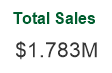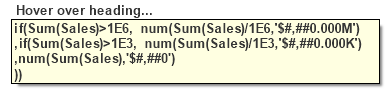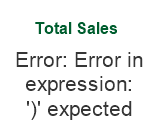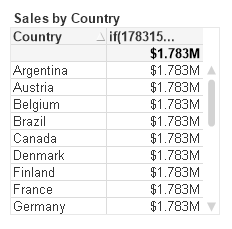Scaling Numbers and DSE Tips

Summary: I demonstrate a simple reusable expression to auto scale numbers in QlikView. This leads to an exploration of some of the finer details of dollar sign expansion.

A QVW example to accompany this post is available for download at http://qlikviewcookbook.com/wp-content/uploads/2016/05/ScalingNumbers.qvw.

The QlikView auto-scaling feature selects an appropriate unit – billion, million, thousands — based on the magnitude of the  Y-axis values.  It’s a nice feature  available in Line and Bar charts.  How can we create the same functionality in Text Objects or Straight Tables?

It’s easy enough to use an if() function that tests the magnitude, does any necessary division, and formats appropriately. For example:

if(Sum(Sales)>1E6, num(Sum(Sales)/1E6,'\$#,##0.000M')
,if(Sum(Sales)>1E3, num(Sum(Sales)/1E3,'\$#,##0.000K')
,num(Sum(Sales),'\$#,##0')
))

(The 1E6 is an easier way to write 1000000).

To avoid repeated coding of “Sum(Sales)” I create a reusable variable with parameters in the script like this:

SET vScaleNumber=if(\$1>1E6, num(\$1/1E6,'\$#,##0.000M')
,if(\$1>1E3, num(\$1/1E3,'\$#,##0.000K')
,num(\$1,'\$#,##0')
));

Now I can use the variable vScaleNumber in a Text Object as:

=\$(vScaleNumber(Sum(Sales)))

The string “Sum(Sales)” will get substituted in  every occurrence of “\$1”.  I ‘ll get an appropriately formatted number like:If I use “\$(vScaleNumber(Sum(Sales)))” in a Straight Table expression without label, hovering over the column heading will show me the full substitution in  a tooltip.I  can see that the “\$1” substitution occurs before the expression is evaluated, and the substituted expression looks like:

if(Sum(Sales)>1E6, num(Sum(Sales)/1E6,'\$#,##0.000M')
,if(Sum(Sales)>1E3, num(Sum(Sales)/1E3,'\$#,##0.000K')
,num(Sum(Sales),'\$#,##0')
))

I’ve avoided re-typing “Sum(Sales)”. But I may have a concern about the performance implications of repeated execution of “Sum(Sales)”.  And what about more complex expressions such as “Round(Sum(Sales),10)”?  The comma in that expression will break the syntax as variable parameters always treat commas as parameter separators.I can fix the comma/performance problem by using Dollar Sign Expansion (DSE) with an “=”.  The “=” will cause the expression to evaluate and pass the numerical result to vScaleNumber.

=\$(vScaleNumber(\$(=round(Sum(Sales),10))))

Checking the expansion in a Straight Table shows:

if(1783150>1E6, num(1783150/1E6,'\$#,##0.000M')
,if(1783150>1E3, num(1783150/1E3,'\$#,##0.000K')
,num(1783150,'\$#,##0')
))

I  see the value of “round(Sum(Sales),10)” has been calculated as “1783150”,  yielding an efficient and syntactically correct expression.

Next  I’ll add a Dimension to the Straight Table.  The row results are incorrect!The “=” in the DSE caused the Sum expression  to be evaluated only once for the entire chart, yielding the same value for every row.  How to fix?

I will calculate the sum() expression in a n Expression n column, and then hide this column on the Presentation tab. I can then refer to the hidden column:

=\$(vScaleNumber(Column(1)))

Once again, the expansion yields an efficient and syntactically correct expression.

if(Column(1)>1E6, num(Column(1)/1E6,'\$#,##0.000M')
,if(Column(1)>1E3, num(Column(1)/1E3,'\$#,##0.000K')
,num(Column(1),'\$#,##0')
))

I started this post by demonstrating a simple expression to format scaled numbers. It’s a function I frequently use.

For more on DSE, see Henric’s post at https://community.qlik.com/blogs/qlikviewdesignblog/2013/11/18/dollar-expansions

A QVW example to accompany this post is available for download at http://qlikviewcookbook.com/wp-content/uploads/2016/05/ScalingNumbers.qvw.

-Rob

7 thoughts on “Scaling Numbers and DSE Tips”

1.Christophe Brault says:

Hi,

First thanks for this great post Rob, i wasn’t using this tip to scale number. when necessary, i do this by a list where user select M,K or \$.

DSE is also great and i’ll try to use it to optimize my app.

My only question is about unicity in the app, because with yourfunction, this different scale level in the same chart.

Don’t you think that it can be confusing ?

And it’s not working in a bar chart …

Thanks again for your work

Christophe

1.Rob Wunderlich says:

I’ll admit that it was a poor example showing different scales in different rows. I wouldn’t do that in practice, I was only trying to move my first example into a DSE discussion.

With a bar chart, you can use the auto-scaling of y-axis available on the Numbers tab. When needed for popups or other text, you can use the hidden column trick. A hidden column in a bar chart has all the Display Options unchecked for the Expression. For an example of a hidden bar column, see Qlikview Cookbook: Tutorial – About Column Visibility http://qlikviewcookbook.com/recipes/download-info/tutorial-about-column-visibility/

1.Christophe Brault says:

Yes !

I didn’t know this tips for hidden bar chart, its useful.

Thanks again, i’ll use it right now

2.Gysbert says:

I usually use the Pick function instead of nested If’s:

pick(RangeMax(1,Ceil(Log10(Fabs(\$1))/3)),
num(\$1,’\$#,##0′),
num(\$1/1E3,’\$#,##0.000K;-\$#,##0.000K’),
num(\$1/1E6,’\$#,##0.000M;-\$#,##0.000M’),
num(\$1/1E9,’\$#,##0.000B;-\$#,##0.000B’)
)

As you see it does need a bit of trickery to deal with 0 and negative values.

1.Gysbert says:

and of course num(\$1,’\$#,##0′) should be num(\$1,’\$#,##0;-\$#,##0′),

3.Karl says:

Great post Rob.

4.Manish Kachhia says:

I think this should be Sum(Sales)>=1E6 and Sum(Sales)>=1E3
I mean >= instead of only >